Question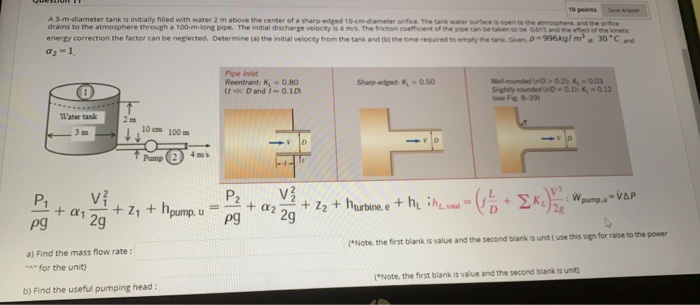We will take the point 1 at the free surface of the tank and point 2 at the exit of the pipe. after this centerline is taken at the centerline of the orific where Z2=0. Positive direction of z is taken upwards

Fluid velocity at free surface is very low and almost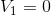Also the fluid at both the points is open to atmosphere so in that case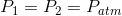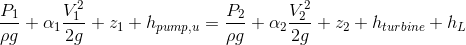After putting the above mentioned conditions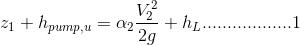where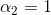Given

So from here the total head can be defined as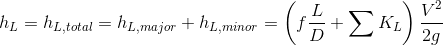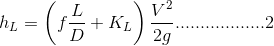Since the dia of the piping system is constant and the initial velocity is given as 4 m/s so from here required mass flow rate and head can be calculated as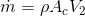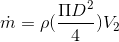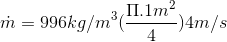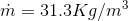Answer

Now from equation 1 and 2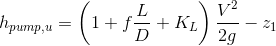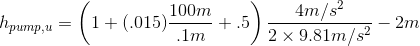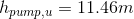Answer

#### Earn Coins

Coins can be redeemed for fabulous gifts.

Similar Homework Help Questions
• ### A 3-m-diameter tank is initially filled with water 2 m above the center of a sharp-edged 10-cm-diameter orifice. The tank water surface is open to the atmosphere, and the orifice drains to the at...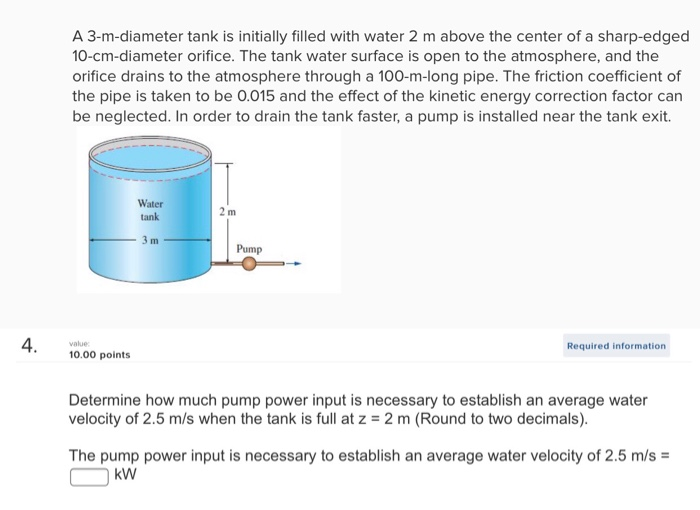A 3-m-diameter tank is initially filled with water 2 m above the center of a sharp-edged 10-cm-diameter orifice. The tank water surface is open to the atmosphere, and the orifice drains to the atmosphere through a 100-m-long pipe. The friction coefficient of the pipe is taken to be 0.015 and the effect of the kinetic energy correction factor can be neglected. In order to drain the tank faster, a pump is installed near the tank exit. Water tank Pump Required...

• ### A 3-m-diameter tank is initially filled with water 2 m above the center of a sharp-edged 10-cm-diameter orifice. The tank water surface is open to the atmosphere, and the orifice drains t...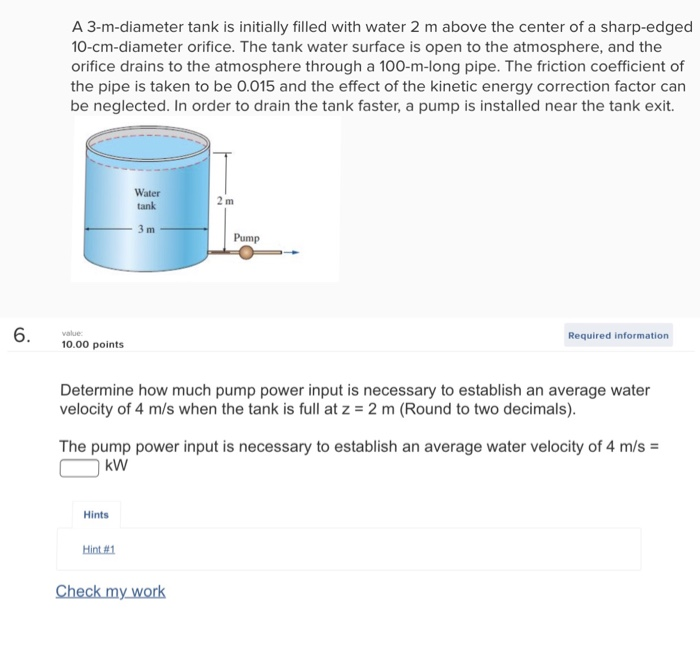A 3-m-diameter tank is initially filled with water 2 m above the center of a sharp-edged 10-cm-diameter orifice. The tank water surface is open to the atmosphere, and the orifice drains to the atmosphere through a 100-m-long pipe. The friction coefficient of the pipe is taken to be 0.015 and the effect of the kinetic energy correction factor can be neglected. In order to drain the tank faster, a pump is installed near the tank exit. Water tank Pump 6...

• ### A 3-m-diameter tank is initially filled with water 2 m above the center of a sharp-edg...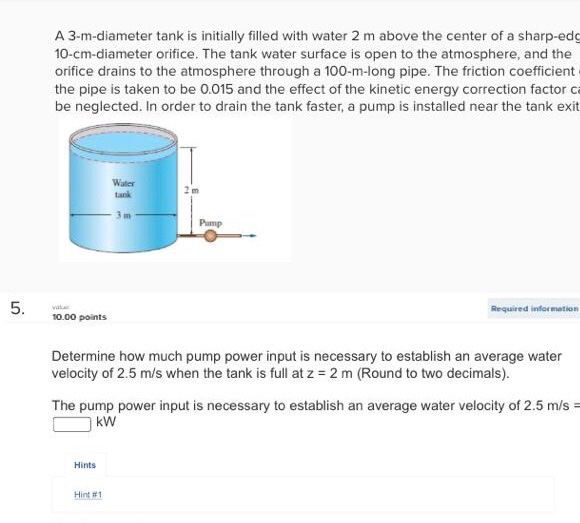A 3-m-diameter tank is initially filled with water 2 m above the center of a sharp-edg 10-cm-diameter orifice. The tank water surface is open to the atmosphere, and the orifice drains to the atmosphere through a 100-m-long pipe. The friction coefficient the pipe is taken to be 0.015 and the effect of the kinetic energy correction factor ca be neglected. In order to drain the tank faster, a pump is installed near the tank exit Water tank Pamp Required infor...

• ### 2. Find the time taken by water for completely emptying to tank. Take C 0.6 12 ft 10 ft Water 4-diameter, sharp-edg...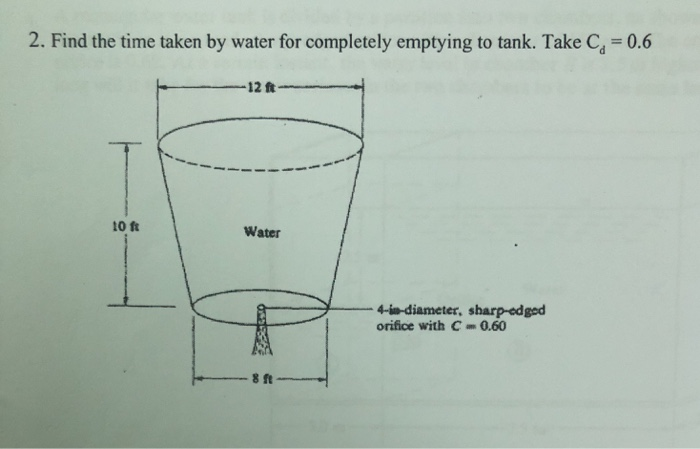2. Find the time taken by water for completely emptying to tank. Take C 0.6 12 ft 10 ft Water 4-diameter, sharp-edged orifice with C 0.60

• ### Problem 4 (50 points) water is discharged from a reservoir at a rate of 18.103 m2/s using two horizontal cast iron pipes nected in series and a pump between them. The first pipe is 20 m long and...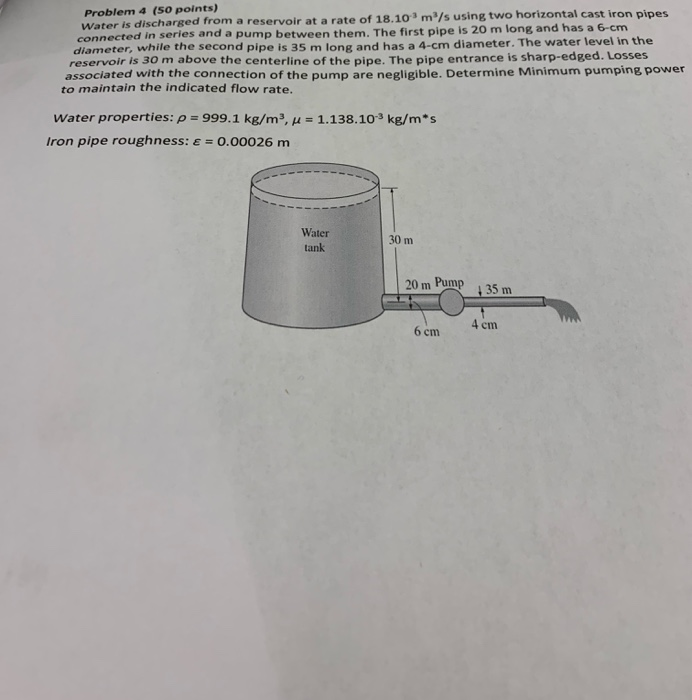Problem 4 (50 points) water is discharged from a reservoir at a rate of 18.103 m2/s using two horizontal cast iron pipes nected in series and a pump between them. The first pipe is 20 m long and has a 6-cm diameter, while the second pipe is 35 m long and has a 4-cm diameter. The water level in the reservoir is 30 m above the centerline of the pipe. The pipe entrance is sharp-edged. Losses associated with the connection...

• ### fluid mechanics ASAP please Problem 4 (50 points) Water is discharged from a reservoir at a rate of 18.103 m3/s using two horizontal cast iron pipes connected in series and a pump between them. T...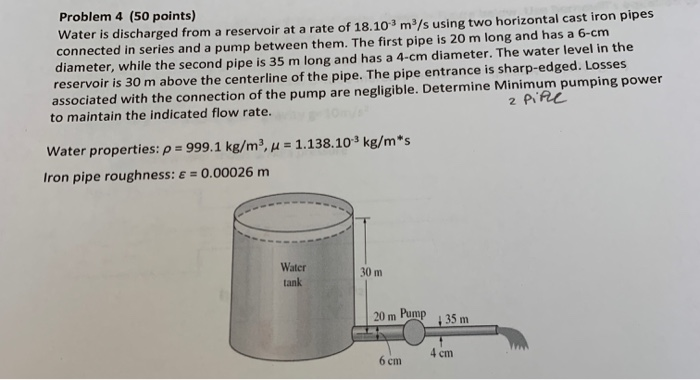fluid mechanics ASAP please Problem 4 (50 points) Water is discharged from a reservoir at a rate of 18.103 m3/s using two horizontal cast iron pipes connected in series and a pump between them. The first pipe is 20 m long and has a 6-cm diameter, while the second pipe is 35 m long and has a 4-cm diameter. The water level in the reservoir is 30 m above the centerline of the pipe. The pipe entrance is sharp-edged. Losses...

• ### A water tank filled with solar-heated water at 40°C is to be used for showers in...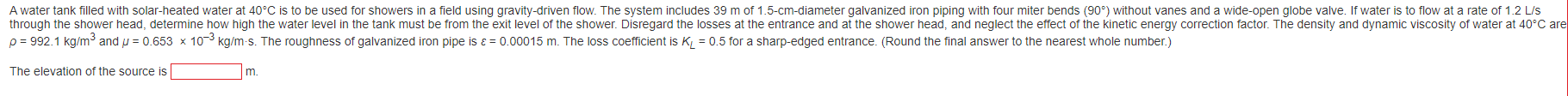A water tank filled with solar-heated water at 40°C is to be used for showers in a field using gravity-driven flow. The system includes 39 m of 1.5-cm-diameter galvanized iron piping with four miter bends (90°) without vanes and a wide-open globe valve. If water is to flow at a rate of 1.2 L/S through the shower head, determine how high the water level in the tank must be from the exit level of the shower. Disregard the losses at...

• ### Water, with density of 1000 kg/m3 and kinematic viscosity of 1.0 x 10- m2/s, is pumped through a ...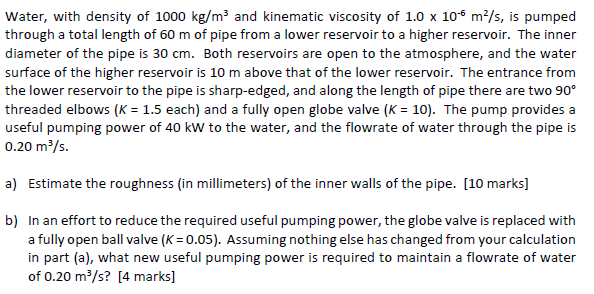Water, with density of 1000 kg/m3 and kinematic viscosity of 1.0 x 10- m2/s, is pumped through a total length of 60 m of pipe from a lower reservoir to a higher reservoir. The inner diameter of the pipe is 30 cm. Both reservoirs are open to the atmosphere, and the water surface of the higher reservoir is 10 m above that of the lower reservoir. The entrance from the lower reservoir to the pipe is sharp-edged, and along the...

• ### Two water reservoirs A and B are connected to each other through a 35m-long, 2-cm-diameter cast...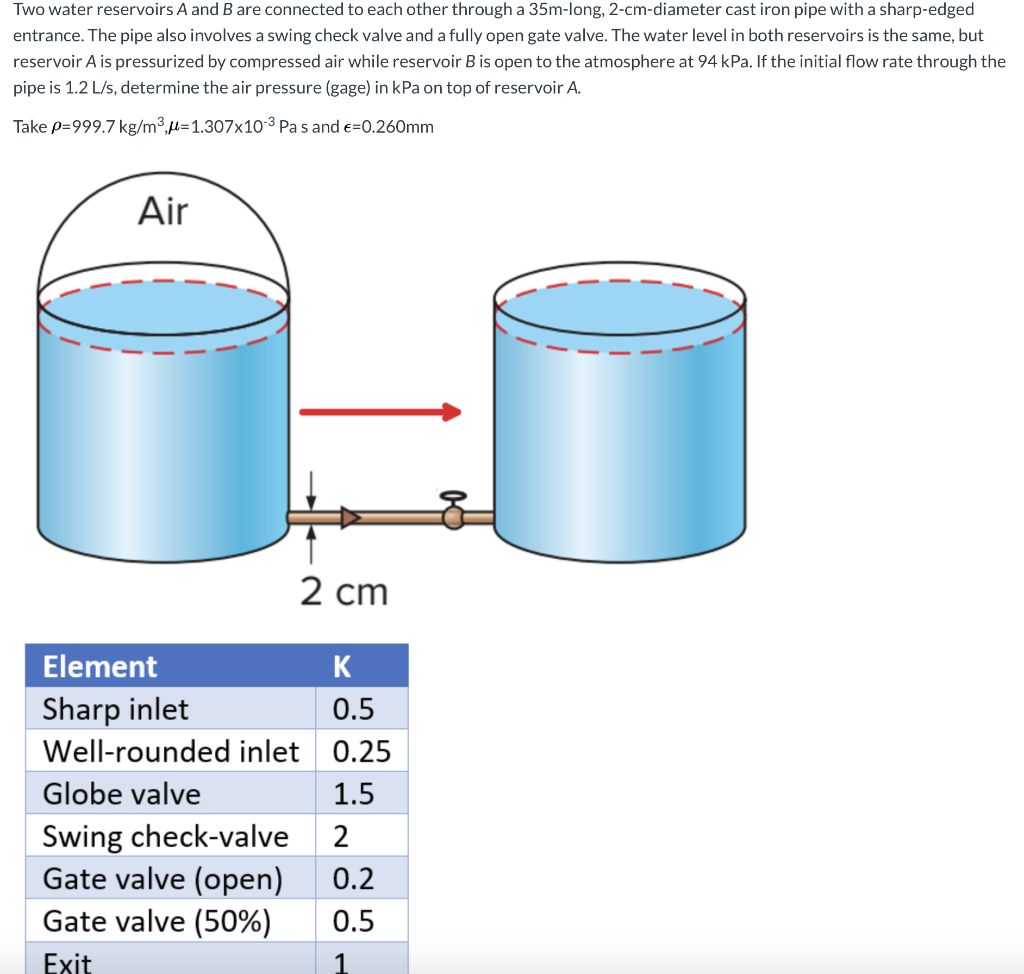Two water reservoirs A and B are connected to each other through a 35m-long, 2-cm-diameter cast iron pipe with a sharp-edged entrance. The pipe also involves a swing check valve and a fully open gate valve. The water level in both reservoirs is the same, but reservoir A is pressurized by compressed air while reservoir B is open to the atmosphere at 94 kPa. If the initial flow rate through the pipe is 1.2 L/s, determine the air pressure (gage)...

• ### Can I know full answer 2. Pressurized tank of water has a 10-cm-diameter orifice at the...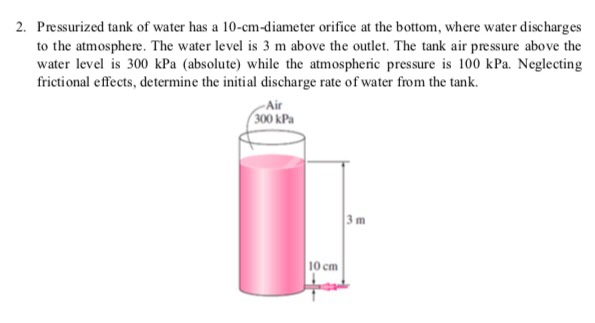Can I know full answer 2. Pressurized tank of water has a 10-cm-diameter orifice at the bottom, where water discharges to the atmosphere. The water level is 3 m above the outlet. The tank air pressure above the water level is 300 kPa (absolute) while the atmospheric pressure is 100 kPa. Neglecting frictional effects, determine the initial discharge rate of water from the tank. Air 00 kPa 3 m 10 cm